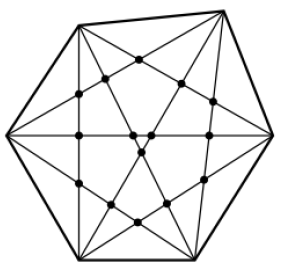### COCI 2006/2007, Contest #2

Consider a convex polygon with N vertices, with the additional property that no three diagonals intersect in a single point. Find the number of intersections between pairs of diagonals in such a polygon.

The figure below shows one such polygon with 6 vertices.Note: a polygon is convex if all of its interior angles are less than 180 degrees.

### Input

The first and only line of input contains a single integer N (3 ≤ N ≤ 100).

### Output

Output the number of intersections on a single line.

```3
```

```0
```

```4
```

```1
```

```6
```

### Output

```15
```

Point Value: 7
Time Limit: 1.00s
Memory Limit: 32M